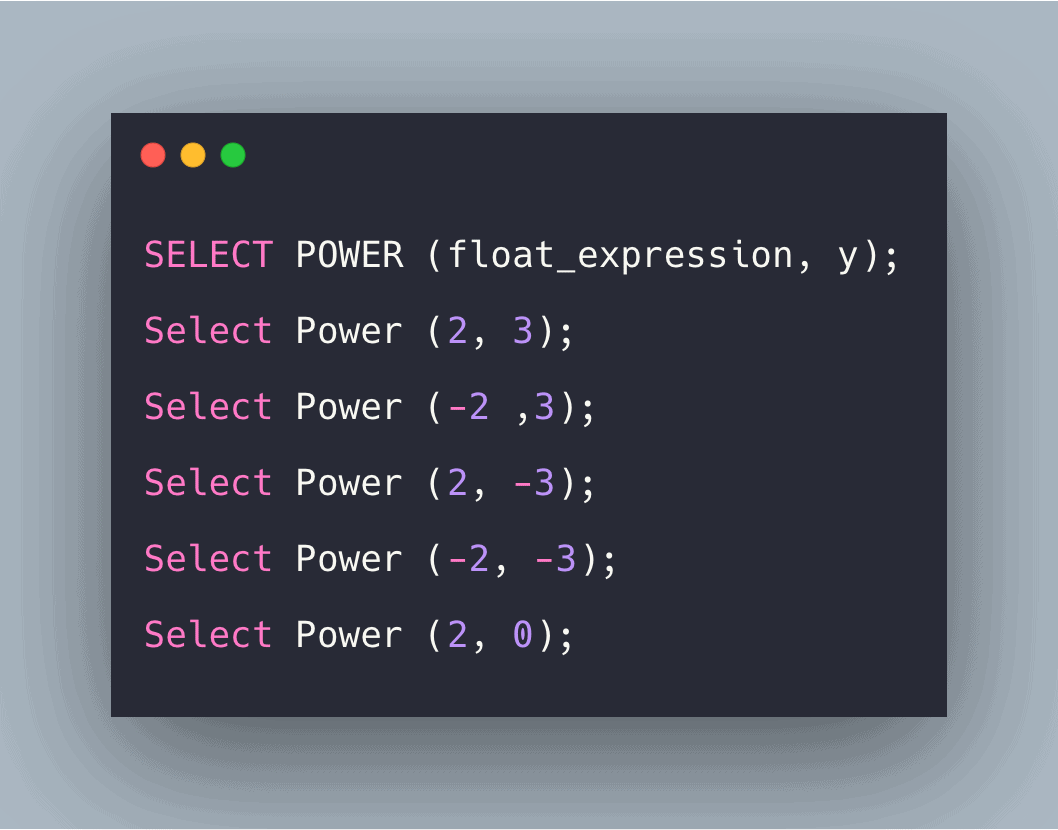# SQL Power() Function: Complete Guide

0
6The SQL DISTINCT command with the SQL POWER() function can be used to retrieve unique data depending on a specified expression.

## SQL POWER()

SQL POWER() is a built-in function used to return the value of the specified expression to the specified power. SQL POWER function is used to return the value of the determined expression to the specified power. SQL POWER() method returns the value of a number raised to another, where both of the numbers are passed as arguments.

### Syntax

```SELECT POWER (float_expression, y);
```

### Parameters

1. Float_expression: This is the expression whose value is to be calculated raised to absolute power, and that expression can be implicitly converted to float.
2. Y: The value of the power. It can be an expression of exact numeric datatype except for bit datatype. If the value of power is negative, then 0 will be returned.

### Examples

#### Query 1

```Select Power (2, 3);
```

`8`

#### Explanation

Here, 2 is the expression, and 3 is the power.

#### Query 2

```Select Power (-2 ,3);
```

`-8`

#### Explanation

Here, the value of the negative expression is calculated where -2 is the expression and 3 is the power.

#### Query 3

```Select Power (2, -3);
```

`0`

#### Explanation

Here, the value of power was negative. So, 0 was returned.

#### Query 4

`Select Power (-2, -3);`

`0`

#### Explanation

Here, 0 is returned as the value of power is negative. It does not matter whether the float expression is negative or not.

#### Query 5

```Select Power (2, 0);
```

`1`

#### Explanation

Here, the power value is 0, and any expression whose power is 0 returns value 1.

#### Query 6

```Select Power (2, 1);
```

`2`

#### Explanation

Here, the value of power is 1. So, any value whose power is raised to 1 returns the same value.

#### Query 7

```Select Power (5*2, 2);
```

`100`

#### Explanation

Here, the value of the arithmetic expression can also be calculated. So, (5*2) expression, which results in 10 when raised to power 2, gave the appropriate result.